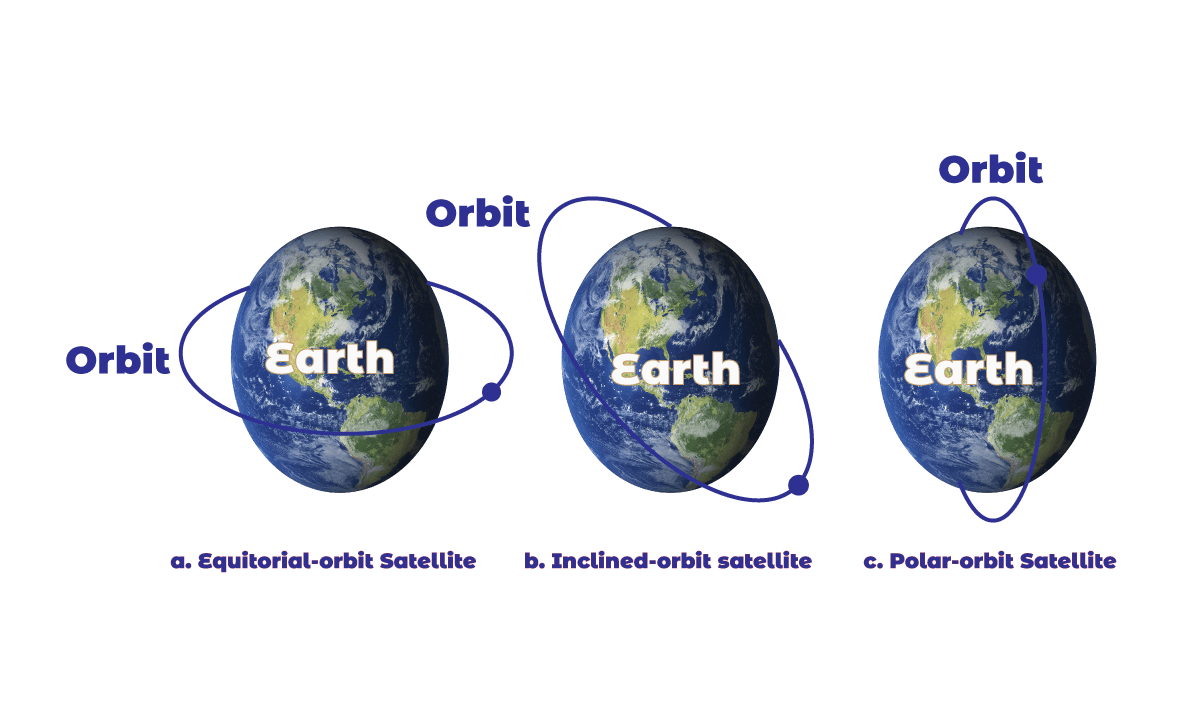## Satellite Networks:

Satellite networks is a combination of nodes that provides communication from one point on the Earth to another. A node is a network that can be a satellite, an Earth station, or an end-user terminal or telephone. Although a natural satellite, such as the moon, can be used as a relaying node in the network, the use of artificial satellites is preferred because we can install electronic equipment on the satellite to regenerate the signal that has lost its energy during travel. Another restriction on using natural satellites is their distances from the Earth, which creates a long delay in communication.

Satellite networks are like cellular networks in that they divide the planet into cells. Satellites can provide transmission capability to and from any location on Earth.

## Operation of Satellites:

Let us discuss some general issues related to the operation of satellites:

• Orbits: An artificial satellite needs to have an orbit, the path in which it travels around the Earth. The period of the satellite, the time required for a satellite to make complete trips around the earth which defines the period as a function of the distance of the satellite from the center of the Earth.Figure: Satellite Orbits
• Footprint: Satellites process microwaves with bidirectional antennas (line-of-sight). Therefore, the signal from a satellite is normally at a specific area called the footprint. The signal power at the center of the footprint is maximum. The power decreases as we move out from the footprint center. The boundary of the footprint is the location where the power level is at a predefined threshold.
• Frequency Bands for Satellite Communication: The frequencies reserved for satellite microwave communication are in the gigahertz (GHz) range. Each satellite sends and receives over two different bands. Transmission from the Earth to the satellite is called uplink. Transmission from the satellite to the Earth is called the downlink.

## Three categories of Satellites:

Based on the location of the orbit, the satellite networks can be divided into three categories which are briefly discussed below:

i. GEO Satellites: Line-of-sight requires that the sending and receiving antennas be locked onto each other’s location at all times (one antenna must have the other sight). For this reason, a satellite that moves faster or slower than the Earth’s rotation is useful only for short periods. To ensure constant communication, the satellite must move at the same speed as the Earth so that it seems to remain fixed to above a certain spot. Such satellites are called geostationary.Figure: Satellites in Geostationary orbit

Because orbital speed is based on the distance from the planet, only one orbit can be geostationary. This orbit occurs at the equatorial plane and approximately 22,000 mi from the surface of the Earth. But one geostationary satellite cannot cover the whole Earth. It takes a minimum of three satellites equidistant from each other in geostationary Earth Orbit (GEO) to provide full global transmission.

ii. MEO Satellites: Medium-Earth-orbit (MEO) satellites are positioned between the two Van Allen belts. A satellite at this orbit takes approximately 6 to 8 hours to circle the Earth.

• Global Positioning System: one example of the MEO satellite system is the Global Positioning System (GPS), contracted and operated by the U.S. Department of Defense, orbiting at an altitude about 18,000 km (11, 000 mi) above the Earth. The system consists of 24 satellites and is used for land, sea, and air navigation to provide time and location for vehicles and ships. A GPS receiver has an almanac that tells the current position of each satellite.
• Trilateration: GPS is based on a principle called trilateration. The terms trilateration and triangulation are normally used interchangeably. We use the word trilateration, which means using three distances, instead of triangulation, which may mean using three angels.
• Measuring the distance: the trilateration principle can find on the Earth if we know our distance from three satellites and know the position of each satellite. The position of each satellite can be calculated by a GPS receiver (using the predetermined path of the satellites). Measuring the distance is done using a principle called one-way ranging. The computer on the receiver measures the delay between signals from the satellites and its copy of the signals to determine the distances to the satellites.
• Synchronization: Satellites use atomic clocks that are precise and can function synchronously with each other. For the moment, let us assume that all GPS satellites and the receiver on the Earth are synchronized. Each of 24 satellites synchronously transmits a complex signal, each satellite’s signal having a unique pattern.

iii. LEO Satellites: Low-Earth-orbit (LEO) satellites have polar orbits. The altitude is between 500 and 2000 km, with a rotation period of 90 to 120 min. The satellite has a speed of 20,000 to 25,000 km/h. LEO system usually has a cellular type of access, similar to the cellular telephone system. The footprint normally has a diameter of 8000 km. Because LEO satellites are close to Earth, the round-trip time propagation delay is normally less than 20 ms, which is acceptable for audio communication.

LEO system made of a constellation of satellites that work together as a network; each satellite acts as a switch. Satellites that are close to each other are connected through inter-satellite links (ISLs). A mobile system communicates with the satellite through a user mobile link (UML). A satellite can also communicate with an Earth station through a gateway link (GWL).

LEO satellites can be divided into three categories: Little LEOs, big LEOs, and broadband LEOs. The little LEOs operate under 1 GHz. They are mostly used for low-date-rate messaging. The big LEOs operate between 1 and 3 GHz. Globalstar is one of the examples of a big LEO satellite system. The broadband LEOs provide communication similar to fiber-optic-networks.

Reference: Data Communications and Networking 5th Edition written by Behrouz A. Forouzan.